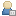###Show Posts

This section allows you to view all posts made by this member. Note that you can only see posts made in areas you currently have access to.

### Messages - Havard

Pages: 1 
16
##### 2D / Support Fullscreen at lower resolutions or make windows scalable
« on: 2009-Aug-06 »
Title says it all really.  For retroremakes it would be helpful to support fullscreen at 320x200, 320x240 etc

or

Could you make windows scalable so that they can be dragged to any size on the desktop?

17
« on: 2009-Aug-06 »
Hi - I'm new to GL too and have found this link which may be helpful:

http://www.miscthings.co.uk/GLBasic

18
##### GLBasic - en / Re: Help for a beginner
« on: 2009-Aug-06 »
Thanks for the replies - I have discoverd that I can only go fullscreen at 640x480 which is a bit of a pain if you are writing retroremakes/games.  I suppose the obvious solution is to double the graphics size (shame you can't resize teh window as this would have the desired effect).

As to my code - you are nearly right as about the jump but I use teh variable p1state to define whether the player has started a move and jumps the control input until this is finished.  So I don't think this is the problem.  I am pretty sure that if p1state=0 the program will continue to move to the check for both key presses and back kick should be started but for some reason it is not registering the key presses but just the punch and then p1state is set.  I cna get it to work by holding both arrow keys first and the hitting cntrl but I don't understand why it doesn't work unless I have got my logic wrong. (I am sure this is how it works in my original blitz version)?

Finally, if you suggest I don't use jumps - what would you suggest I do?  I come from a background of basic with line numbers and got/gosubs were staple program control.  I try to be  abit more modula but struggle!!!

19
##### GLBasic - en / Help for a beginner
« on: 2009-Aug-06 »
Hi

Having frequented retroremakes for a number of years there are a number of familiar names on this site. - so hello old friends!  Having been a Blitz devotee for a number of years, I took up Gernots's offer of an education licence for my school and have been experimenting with GL basic.  I converted one of my games over without too much trouble and now am reviving an old project - Target Renegade Remix - by starting from scratch and rewriting/reusing old code.  I have hit two problems and both of them are quite major and probably linked to my understanding of GL basic.

1. Fullscreen - I can't run at fullscreen or expand teh window to fullscreen at a resolution of 320x200.  Can go fullscreen at 320x240?  This works in blitz.  Any ideas?

2. I am trying to detect simultaneous button presses for cntrl and an arrow key to produce a back kick but I just can't get it to work.  The code is included below - any suggestions, please let me know (I tried a different key instead of cntrl as I wondered if it was a hardware problem with the keyboard but this made no difference).

Code: (glbasic) [Select]
`// --------------------------------- //// Project: TR Remix// Start: Monday, July 27, 2009// IDE Version: 6.164SETSCREEN 640,480,TRUE//LOADFONT "fonts/zx32colour.png",1//LOADFONT "fonts/zx16colour.png",1//load graphicsLOADSPRITE "backgrounds/level01.png",0LOADSPRITE "backgrounds/bottombar2.png",1//player1LOADANIM "graphics/p1.png",100,32,42 //walkingLOADSPRITE "graphics/shadow1.png",101 //shadowLOADANIM "graphics/p1hit.png",102,56,15 //p1 hit to floorLOADANIM "graphics/p1flyingkick.png",103,40,46 //flying kickLOADANIM "graphics/p1punch.png",104,40,45 //p1punchLOADANIM "graphics/p1floorpunch.png",105,28,30 //p1 floor punchLOADANIM "graphics/p1knee.png",106,32,42 //p1 kneeLOADANIM "graphics/p1bk.png",107,40,45 //p1 back kickLOADSPRITE "graphics/head.png",99//Set up variablesGLOBAL p1x=100,p1y=60,p1f=0,p1dir=1,p1state=0GLOBAL scroll=0,lives=3//main loopWHILE KEY(57)=0//draw background DRAWSPRITE 0,n,0 DRAWSPRITE 1,0,141 FOR n=1 TO lives; DRAWSPRITE 99,n*20,170; NEXT //PRINT "P1",0, 150//a\$=Str(p1score): ss=LEN(a\$): a\$="":FOR n=0 TO 5-ss: a\$=a\$+"0":NEXT: a\$=a\$+Str(p1score)//jf_text (fntzx16, 25, 150, a\$)//jf_text (fntzx16, 205, 150, "P1") keypress() drawsprites() SHOWSCREENWENDFUNCTION keypress:right1=0; left1=0; up1=0; down1=0;IF KEY(205) THEN right1=1IF KEY(203) THEN left1=1IF KEY(200) THEN up1=1IF KEY(208) THEN down1=1//code to make player release punch keyIF KEY(29) THEN b1=1IF KEY(29)=0 THEN b1=0IF b1=1 AND bd=0 THEN punch1=1bd=b1PRINT "punch="+punch1,100,150PRINT "right="+right1,100,160PRINT "left="+left1,100,170PRINT "up="+up1,100,180PRINT "p1state="+p1state,100,190PRINT "p1dir="+p1dir, 20,150PRINT "bd="+bd, 20,160PRINT "b1="+b1, 20,170IF p1state<>0 THEN GOTO jump2//check for back kickIF punch1 AND right1 //AND p1dir=-1 p1state=2 p1f=0 delay=0ENDIFIF punch1 AND left1 //AND p1dir=1 p1state=2 p1f=0ENDIF//check for just a punchIF punch1=1 AND p1state=0 p1state=1 p1f=0ENDIFIF right1=1 p1x=p1x+1 p1dir=1 p1f=p1f+.3ENDIFIF left1=1 p1x=p1x-1 p1dir=-1 p1f=p1f-.3ENDIFIF up1=1 p1y=p1y-1 p1f=p1f+(p1dir*.3)ENDIFIF down1=1 p1y=p1y+1 p1f=p1f+(p1dir*.3)ENDIFIF p1f>11 THEN p1f=0IF p1f<0 THEN p1f=10//limits fo x AND y movement FOR playerIF p1x<8 THEN p1x=8IF p1x>300 THEN p1x=300IF p1y>92 THEN p1y=92IF p1y<42 THEN p1y=42//scroll screen if all baddies beaten in sectionIF p1x>200 AND scroll=1 p1x=200 n=n-1ENDIFjump2:ENDFUNCTION// 0rder sprites from top to bottoomFUNCTION drawsprites:FOR n=42 TO 92IF p1state<>0 THEN GOTO JUMP1 IF p1dir=1 AND p1y=n DRAWSPRITE 101,p1x,p1y+35 DRAWANIM 100,p1f,p1x,p1y ENDIF IF p1dir=-1 AND p1y=n DRAWSPRITE 101,p1x+6,p1y+35 DRAWANIM 100,p1f+12,p1x,p1y ENDIFJUMP1://punch IF p1state=1 AND p1dir=1 AND p1y=n DRAWSPRITE 101,p1x,p1y+35 DRAWANIM 104,p1f,p1x-6,p1y-3 p1f=p1f+.1 IF p1f>3 p1state=0 p1f=0 punch1=0 ENDIF ENDIF IF p1state=1 AND p1dir=-1 AND p1y=n DRAWSPRITE 101,p1x+6,p1y+35 DRAWANIM 104,p1f+7,p1x-4,p1y-3 p1f=p1f+.1 IF p1f>3 p1state=0 p1f=0 punch1=0 ENDIF ENDIF//back kick IF p1state=2 AND p1dir=1 AND p1y=n DRAWSPRITE 101,p1x,p1y+35 DRAWANIM 107,p1f+2,p1x-12,p1y-3  p1f=p1f+.1; IF p1f>1 THEN p1f=1 INC delay, 1 IF p1f=1 AND delay=50 p1state=0 p1f=0 punch1=0 delay=0 ENDIF ENDIF IF p1state=2 AND p1dir=-1 AND p1y=n DRAWSPRITE 101,p1x+4,p1y+35 DRAWANIM 107,p1f,p1x+6,p1y-3 p1f=p1f+.1; IF p1f>2 THEN p1f=2 IF p1f=2 AND delay=50 p1state=0 p1f=0 punch1=0 delay=0 ENDIF ENDIFNEXTENDFUNCTION`
If you want to check the old blitz version then click below: